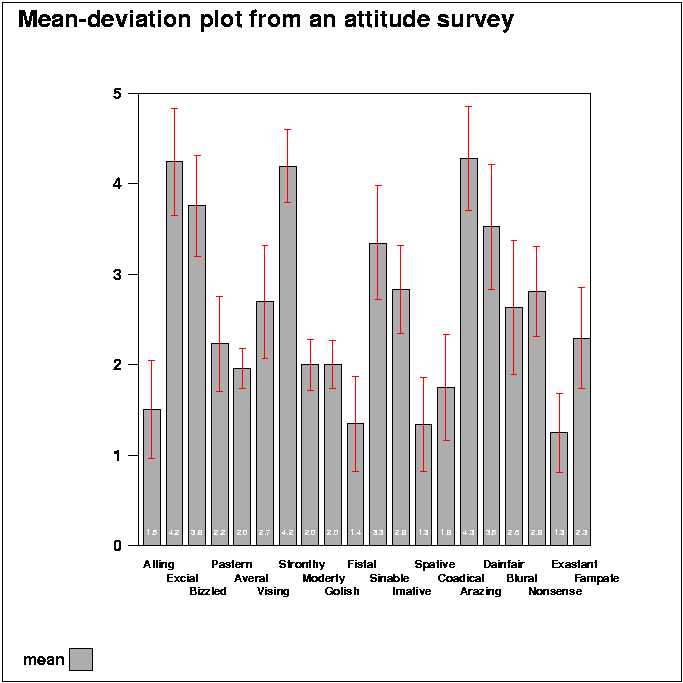# Environment for creative processing of text and numerical data## Mean-deviation plots

S.Mustonen (2004)### Edit field:

```   1 *
2 *Mean-deviation plots are often used for studying results of
3 *attitude surveys. A suitable PLOT scheme for such graphs is created
4 *by a sucro command
5 *
6 */DEVPLOT <data>,<type>
7 *
8 *where <type> is either VBAR or HBAR. The user may enhance the
9 *scheme afterwards according to his/her own requirements.
10 *
11 *The data of 20 variables and 300 observations from an attitude survey
12 *have been generated at random by an |EXAMPLE| sucro, which demonstrates
13 *how to make typical mean-deviation plots.
14 *
15 *.......................................................................
16 *
17 *VARS=ALL / Selecting variables
18 *
19 *Making the mean-deviation graph by the /DEVPLOT sucro:
20 *`/DEVPLOT ATTITUDE,VBAR`
21 *MINSTAT ATTITUDE / saves basic statistics in matrix file STAT.M .
22 *FILE DEL _STATM
23 *FILE SAVE MAT STAT.M TO _STATM / basic statistics to a data file.
24 *up=mean+stddev down=mean-stddev
25 *VAR up,down TO _STATM / computes variables according to above formulas.
26 *
27 *'up' and 'down' variables may be computed also by other formulas.
28 *
29 *`PLOT _STATM` / TYPE=VBAR VARS=CASE,mean SHADING=2 YSCALE=0(1)5
30 *DEV=[RED],down,up        (specifying extra line segments in the graph)
31 *NAMES=[Swissb(7)],3,30   (bar labels on 3 lines with line spacing 30)
32 *
33 *PEN=[BLACK][Swissb(10)][MOVE(0,0)] DEVICE=PS,K.PS
34 *VALUES=[MOVE(-5,0)][Swiss(5)][WHITE],#.#,2
37 *`/GS-PDF K.PS` / Showing the picture by Ghostscript and Acrobat Reader